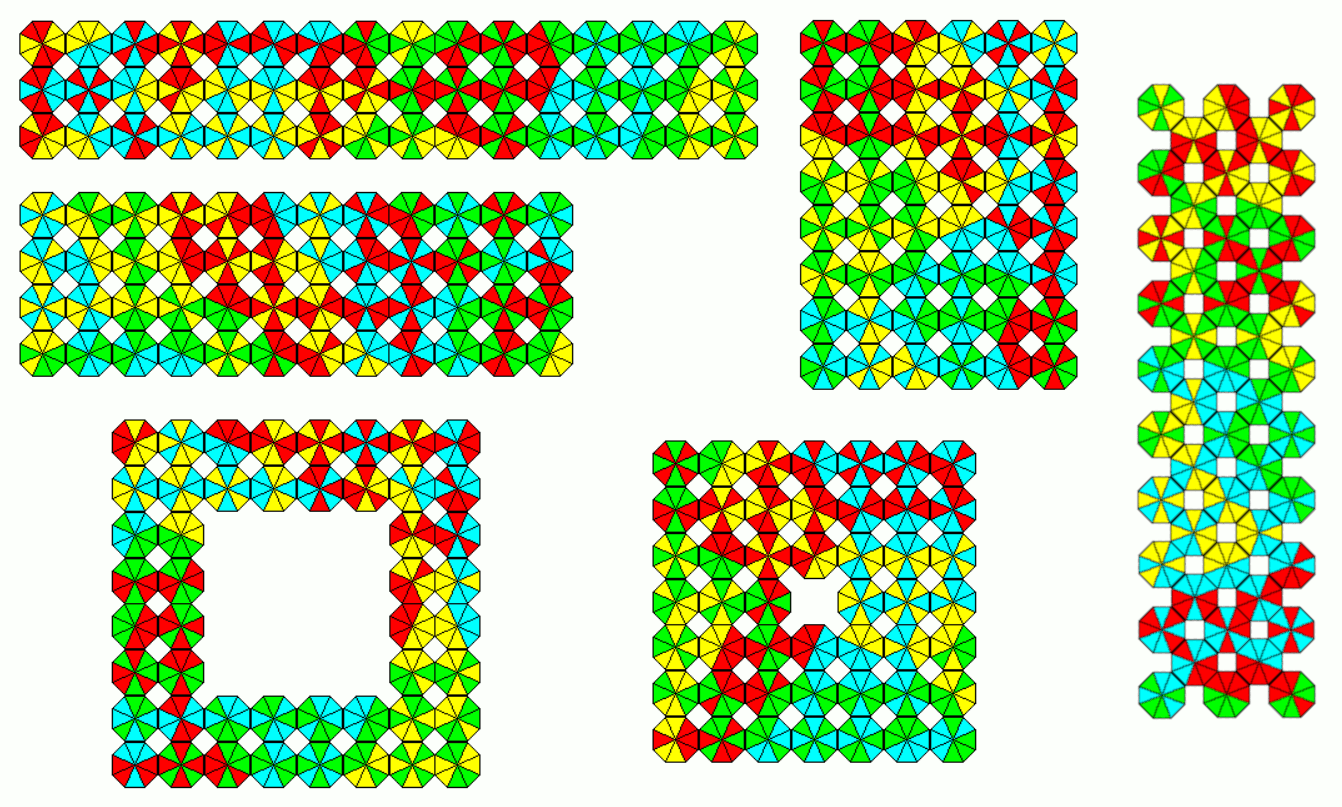## 4 Colors, Exactly 2 Colors on Each piece, One-sided

We have 204 one-sided pieceses and I tried to make rectangles of size 4x51, 6x34 and 12x17. Simple backtracking worked as long as row size was 4 or 6. For the 12x17 retangles I divided the whole area into 3 parts of size 4x17 and filled them using 3 pairs of color combinations (red+lime, lime+yellow), (lime+aqua, aqua+red) and (red+yellow,yellow+aqua). The pieces at the borders must be chosen so that the parts fit together.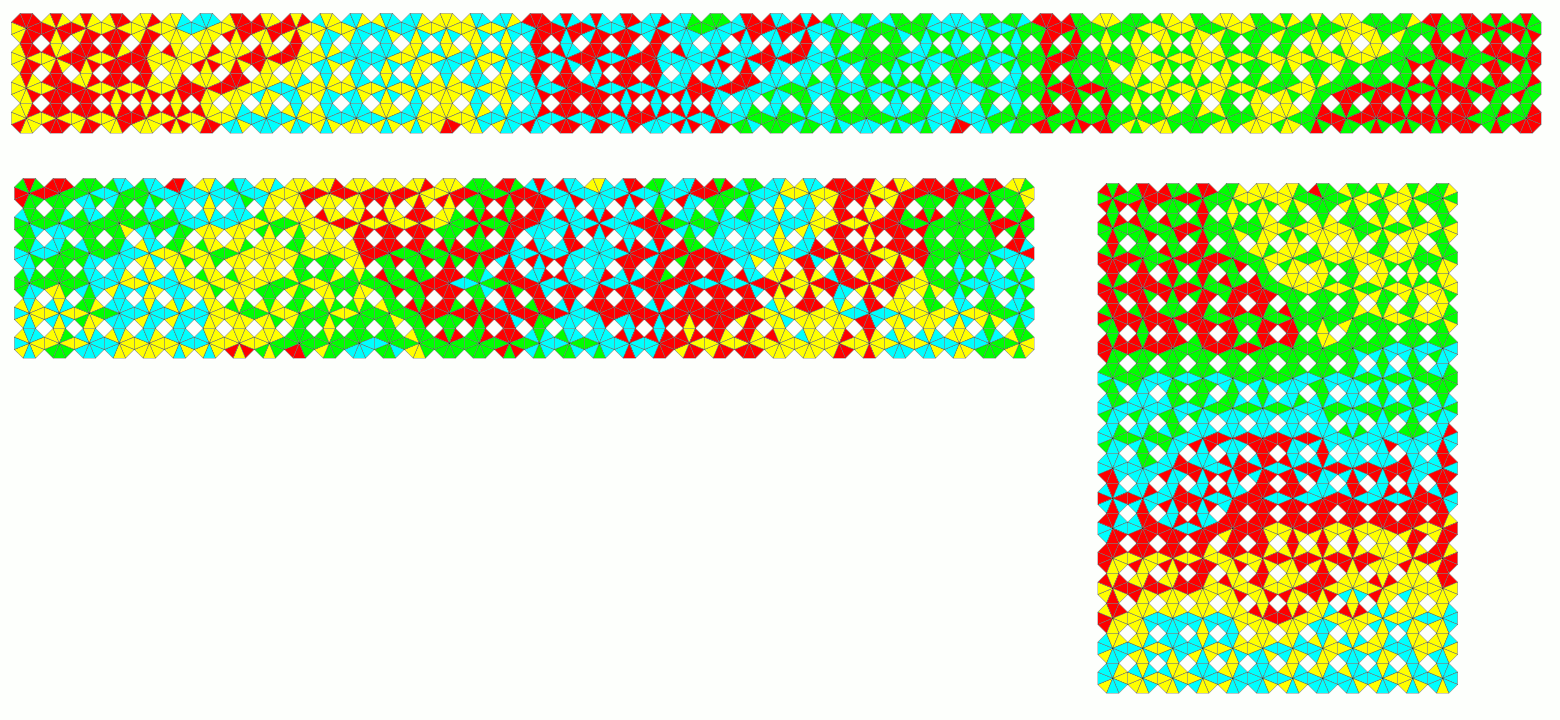## 4 Colors, Exactly 2 Colors on Each piece, Two-sided

There are 168 two-sided pieces which can easily cover rectangles of size 3x56 and 4x42. For the other rectangles 6x28, 7x24, 8x21 and 12x14 I divided the whole area into 3 parts of size 56 or 4 parts of size 28 plus one part of size 56. These parts, not always rectangles, can be filled with pieces of one or two color combinations, respectively. They can be joined to get the whole construction, if the borders are carefully chosen.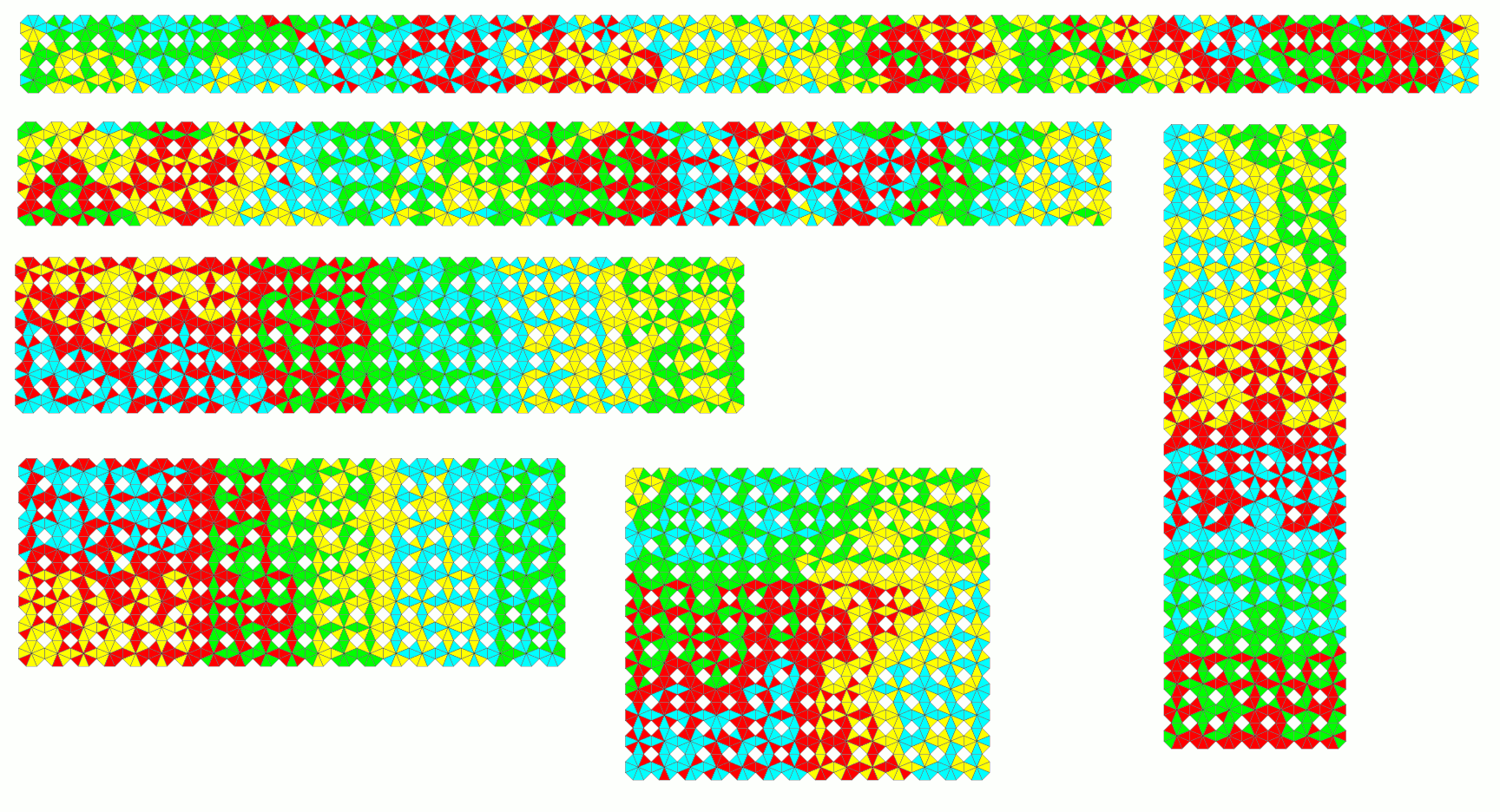## 4 Colors, 1 or 2 Colors on Each piece, One-sided

Adding four same colored pieces to the 204 one-sided pieces with exactly two colors we get 208 one-sided pieces. For each color combination we have 34 pieces. With two of these subsets an area of 68 octagons can be filled. Adding one or two same colored pieces at the corners of such areas we have 69+70+69 pieces enough to solve rectangles of size 4x52, 6x26 and 13x16.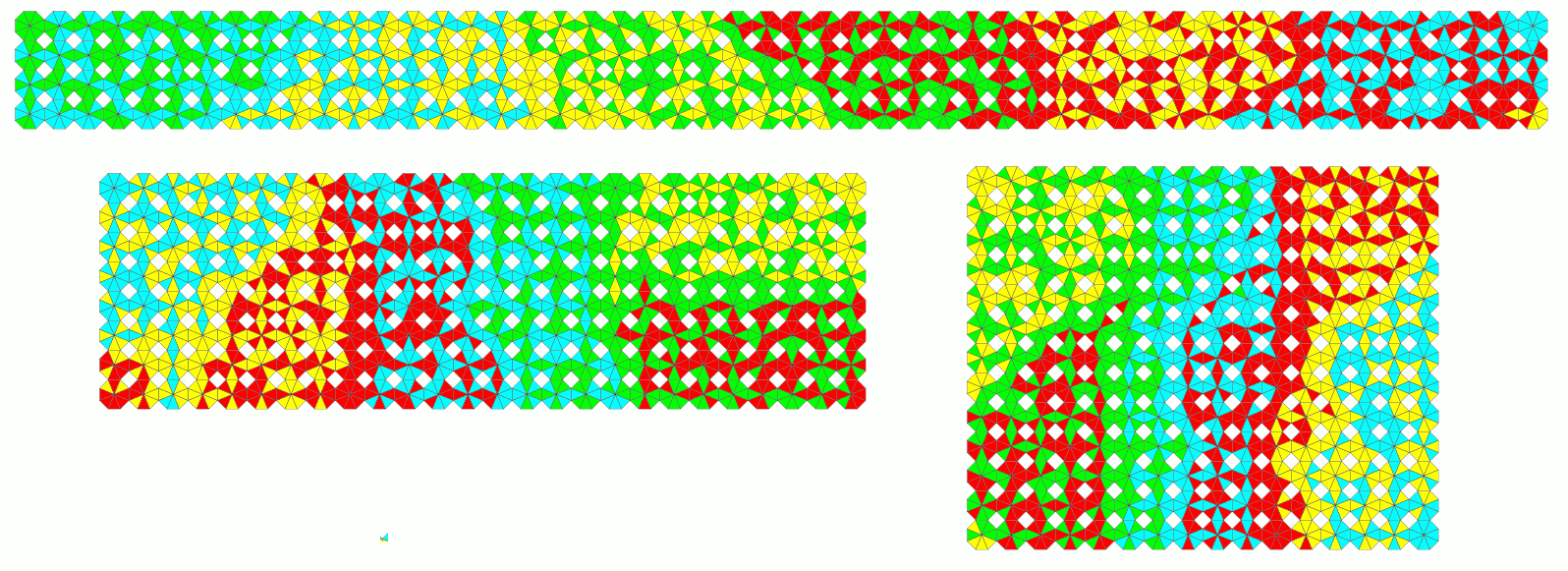## 4 Colors, 1 or 2 Colors on Each piece, Two-sided

There are 168+4=172 two-sided pieces with one or two colors on them. Since 172=4*43 and 42 is prime there is only one interesting rectangle.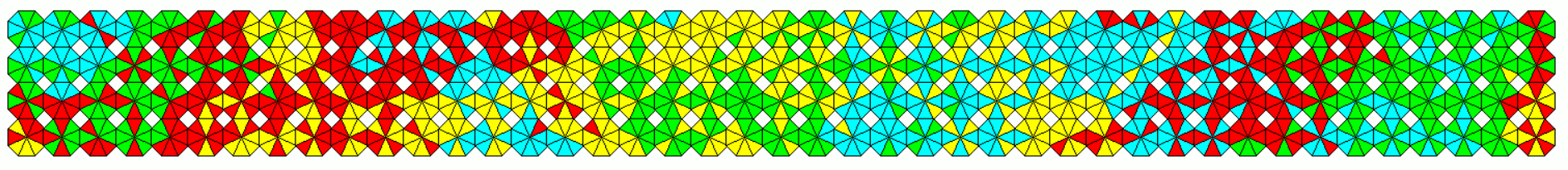## 4 Colors, Ratio of Color Frequencies is 2:6

Four colors are given and we color six edges of the octagons with one color and the remaining two edges with a different one. In this case all possible octagons are symmetric and the the sets of one-sided and two-sided pieces are identical. We have only 48 pieces but it's rather difficult to construct rectangles with color matching edges. Let m be the number of matches for some figures. My counts for m are: 137 for a 3x16 rectangle, 146 for a 4x12 rectangle, 152 for a 6x8 rectangle, 148 for a 7x7 square with a single hole, 124 for a 8x8 square with a 4x4 hole and 144 for a 3x10 jagged rectangle. The probability of a solution by chance is about 0.25^m and the number of arrangements is always less than 48!*8^48. No wonder that the 6x8 rectangle was most difficult to solve. Furthermore backtracking is less effective for longer rows. It seemed to be useful to check in advance if uncovered places in the next row need two colors at most on their edges. Here are the results.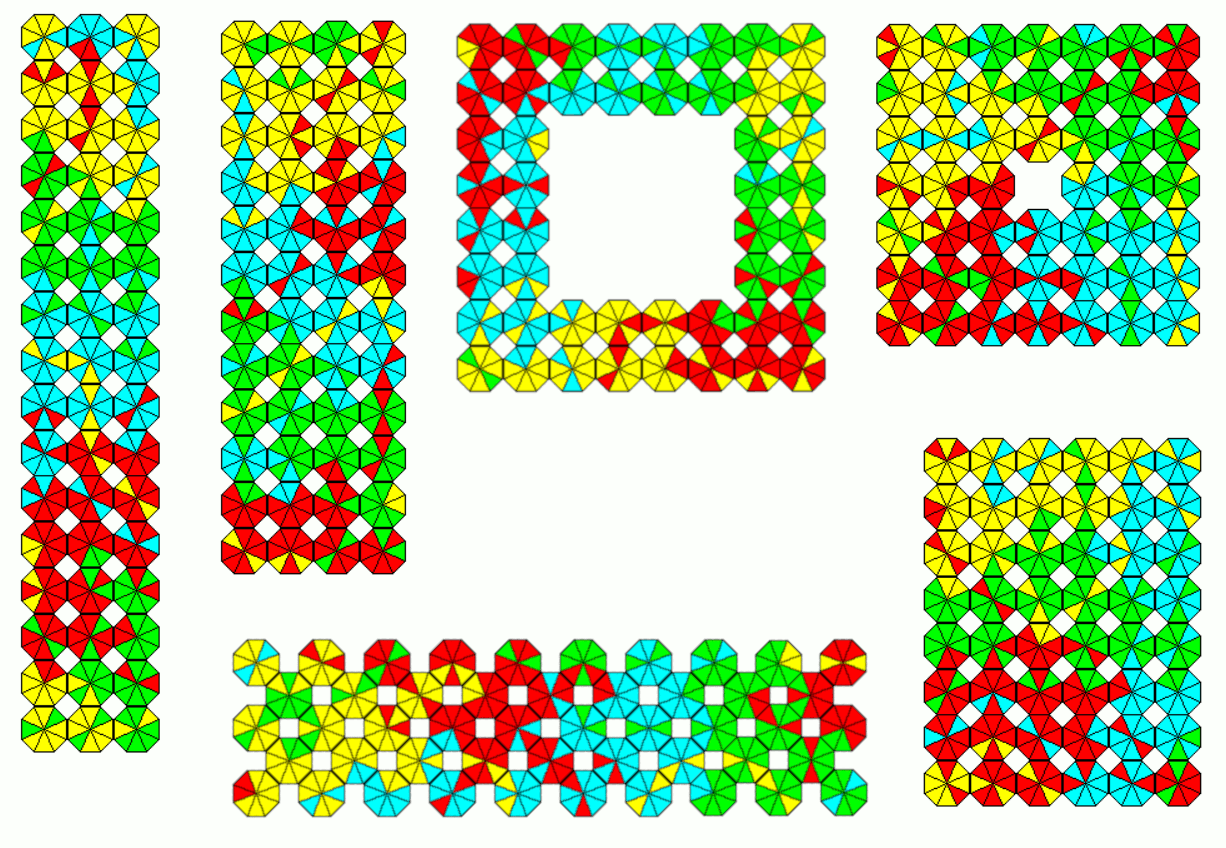## 4 Colors, Ratio of Color Frequencies is 3:5, One-sided

There are 84 one-sided pieces and I solved four rectangles: 3x28, 4x21, 6x14 and 7x12.This was rather easy because the rows for backtracking are small and the number of pieces is not too small.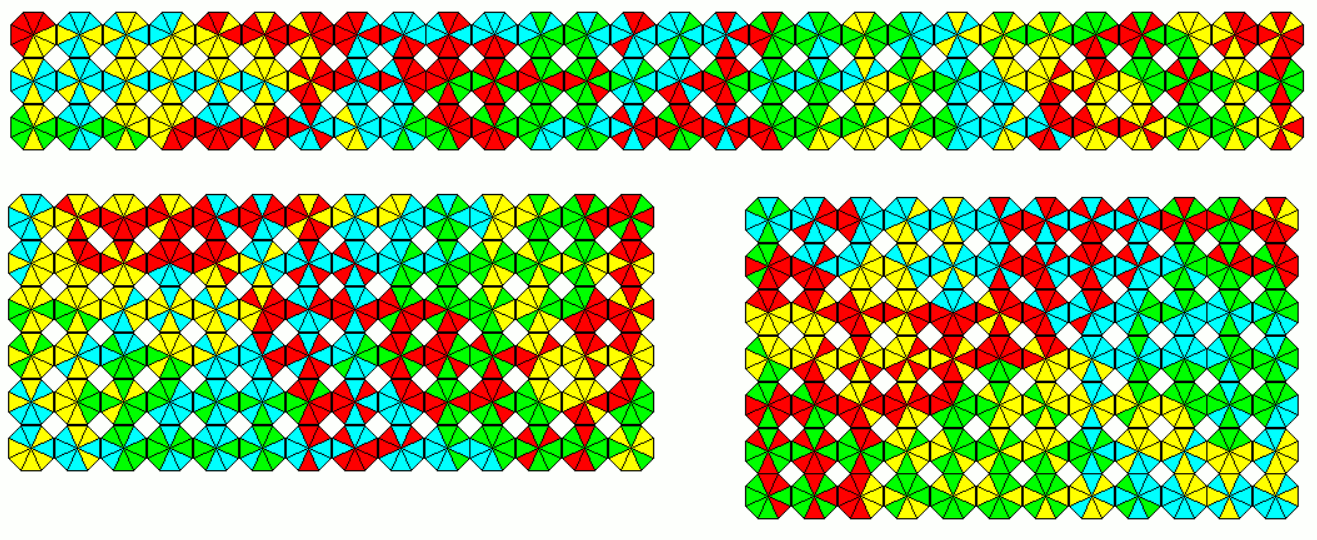## 4 Colors, Ratio of Color Frequencies is 3:5, Two-sided

With the 60 two-sided pieces a lot of constructions can be made because 60 factorizes very well. Beside the rectangles of size 3x20, 4x15, 5x12 and 6x10 I constructed a 8x8 square with a 2x2 hole and two jagged figures.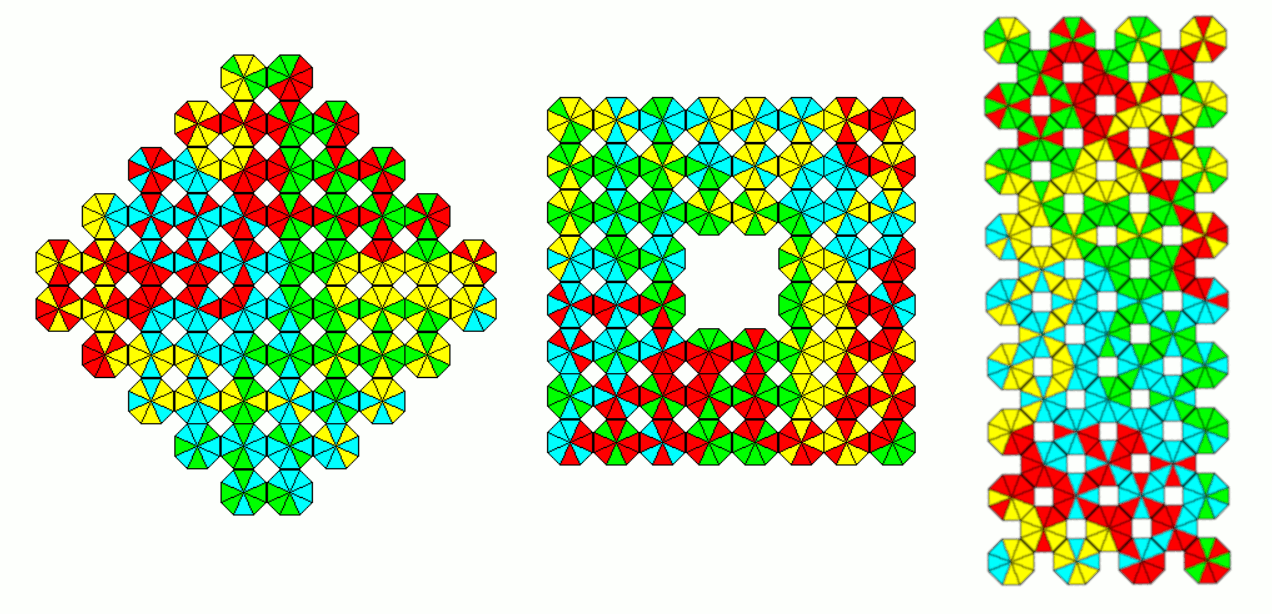## 4 Colors, Ratio of Color Frequencies is 4:4, One-sided

We have 60 one-sided pieces and we can make the same figures, which are already shown using 60 two-sided pieces with color ratio 3:5. The solutions are a little bit harder to get, because the one-sided pieces can't be turned over and there are less possibilities to place the pieces.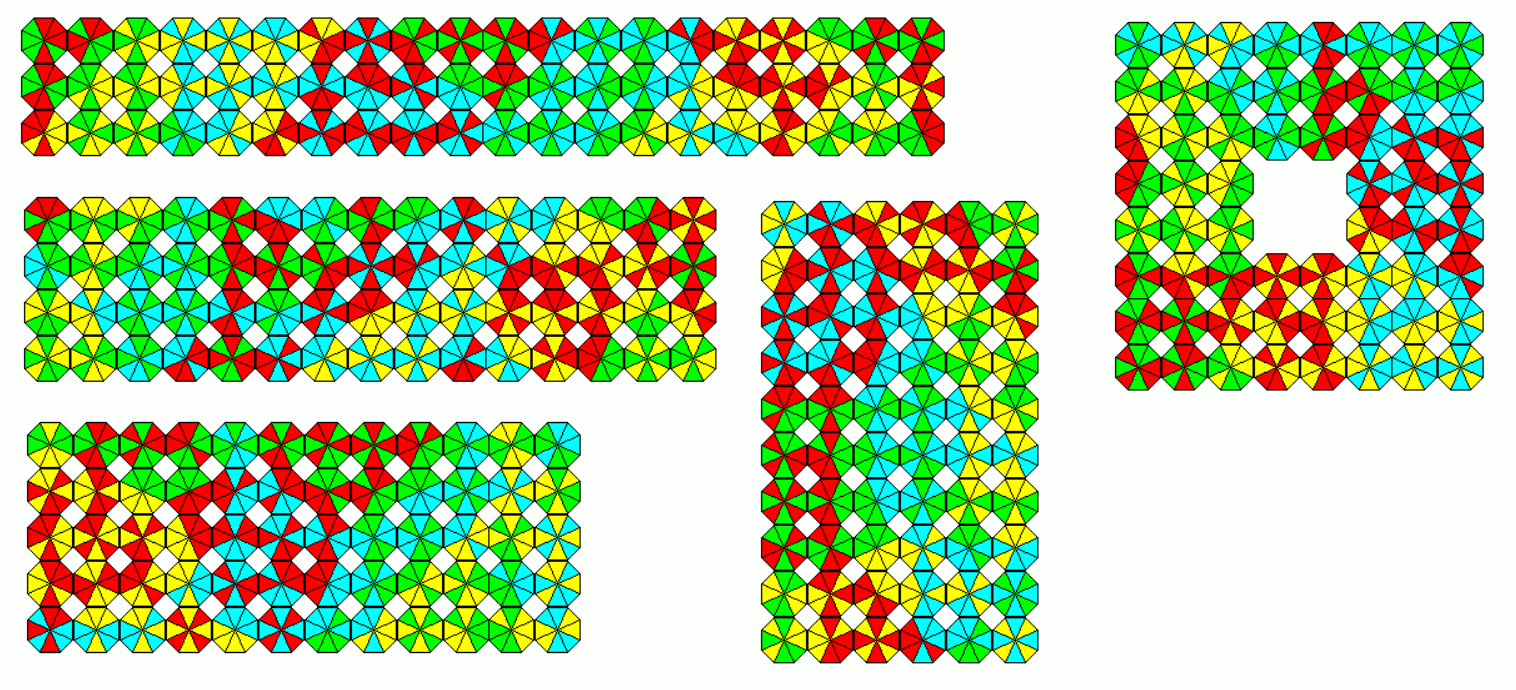## 4 Colors, Ratio of Color Frequencies is 4:4, Two-sided

There are 48 two-sided pieces, but it's harder to make the same figures, which are already shown using the 48 pieces with color ratio 2:6. Almost all of the 4:4 pieces have mirror or rotational symmetry and therefore less possibilties to place them.